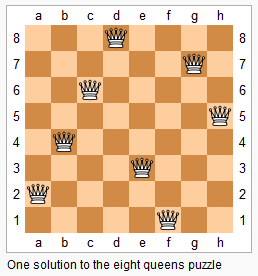# 52. N-Queens II (Hard)

https://leetcode.com/problems/n-queens-ii/

The n-queens puzzle is the problem of placing n queens on an n×n chessboard such that no two queens attack each other.Given an integer n, return the number of distinct solutions to the n-queens puzzle.

Example:

```Input: 4
Output: 2
Explanation: There are two distinct solutions to the 4-queens puzzle as shown below.
[
[".Q..",  // Solution 1
"...Q",
"Q...",
"..Q."],

["..Q.",  // Solution 2
"Q...",
"...Q",
".Q.."]
]
```

## Solutions

``````class Solution {

private Map<String, Boolean> validityMap = new HashMap<>();

int ans = 0;

public int totalNQueens(int n) {
if (n < 1) {
return ans;
}

int[] queens = new int[n];

recurse(queens, 0);

return ans;
}

private void recurse(int[] queens, int col) {
if (col == queens.length) {
ans++;

return;
}

for (int row = 0; row < queens.length; ++row) {
for (int j = col + 1; j < queens.length; j++) {
queens[j] = -1;
}

queens[col] = row;

String key = Arrays.toString(queens);

// We use validityMap to keep the track of valid and invalid sequence so that
// we are able to reduce the computation overhead dramatically.
if (!validityMap.containsKey(key)) {
validityMap.put(key, isValid(queens, col, row));
}

// valid sequence, keep on digging into it.
if (validityMap.get(key)) {
recurse(queens, col + 1);
}
}
}

private boolean isValid(int[] queens, int col, int row) {

// col is the last index, not the length

for (int i = 0; i < col; ++i) {
// previous point (i, queens[i])

// horizontal comparision, check out if any pieces situated on same row
if (queens[i] == row) {
return false;
}

// Assume point A(a1, a2) and B(b1, b2) are diagonally arranged, abs(a1 - b1) == abs(a2 - b2).
// We can imagine that with point A and B as the diagonal points, we can form a square.
// As a square, all the edges should be of same length.

// diagonal comparision
if (Math.abs(col - i) == Math.abs(row - queens[i])) {
return false;
}
}

return true;
}
}
``````